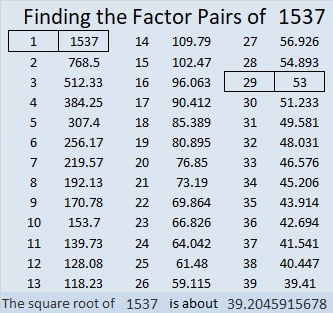# 1537 First Letter of a Secret Message

Contents

### Today’s Puzzle:

The next six puzzles will look like letters of the alphabet, and they will spell out a secret message for you. Will you figure out the message before I have published all of the letters? The difficulty levels of the puzzles will not be in any particular order.### Factors of 1537:

OEIS.org tells us that the largest prime factor of 1537 is 53. Pretty cool!

• 1537 is a composite number.
• Prime factorization: 1537 = 29 × 53.
• 1537 has no exponents greater than 1 in its prime factorization, so √1537 cannot be simplified.
• The exponents in the prime factorization are 1 and 1. Adding one to each exponent and multiplying we get (1 + 1)(1 + 1) = 2 × 2 = 4. Therefore 1537 has exactly 4 factors.
• The factors of 1537 are outlined with their factor pair partners in the graphic below.### More about the Number 1537:

1537 is the DIFFERENCE of two squares in two different ways:
769² – 768² = 1537, and
41² – 12² = 1537.

1537 is also the SUM of two squares in two different ways:
39² + 4² = 1537, and
31² + 24² = 1537.

1537 is the hypotenuse of FOUR Pythagorean triples:
312-1505-1537, calculated from 2(39)(4), 39² – 4², 39² + 4²,
385-1488-1537, calculated from 31² – 24², 2(31)(24), 31² + 24²,
812-1305-1537, which is 29 times (28-45-53), and
1060-1113-1537, which is (20-21-29) times 53.

This site uses Akismet to reduce spam. Learn how your comment data is processed.